Research papers and code for "Chao Yang":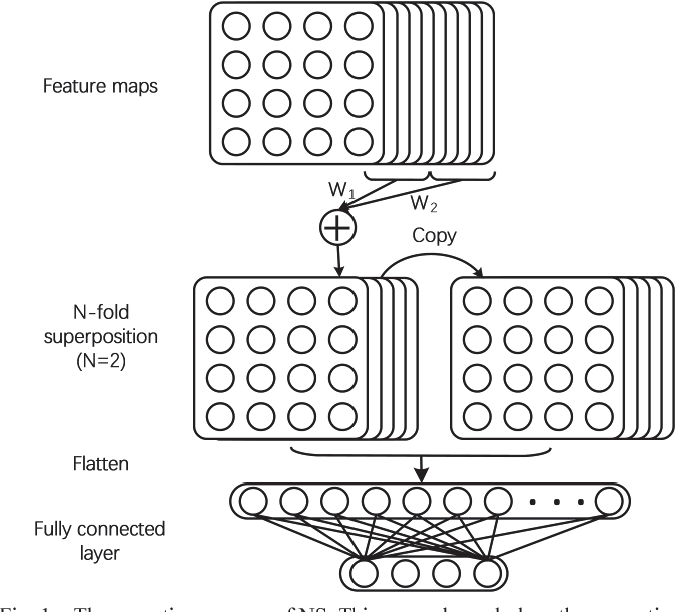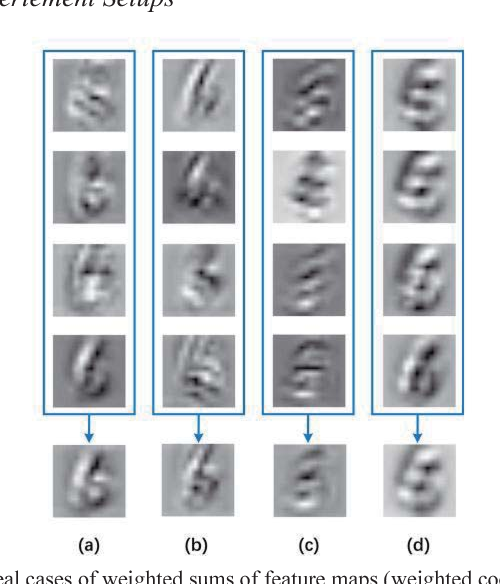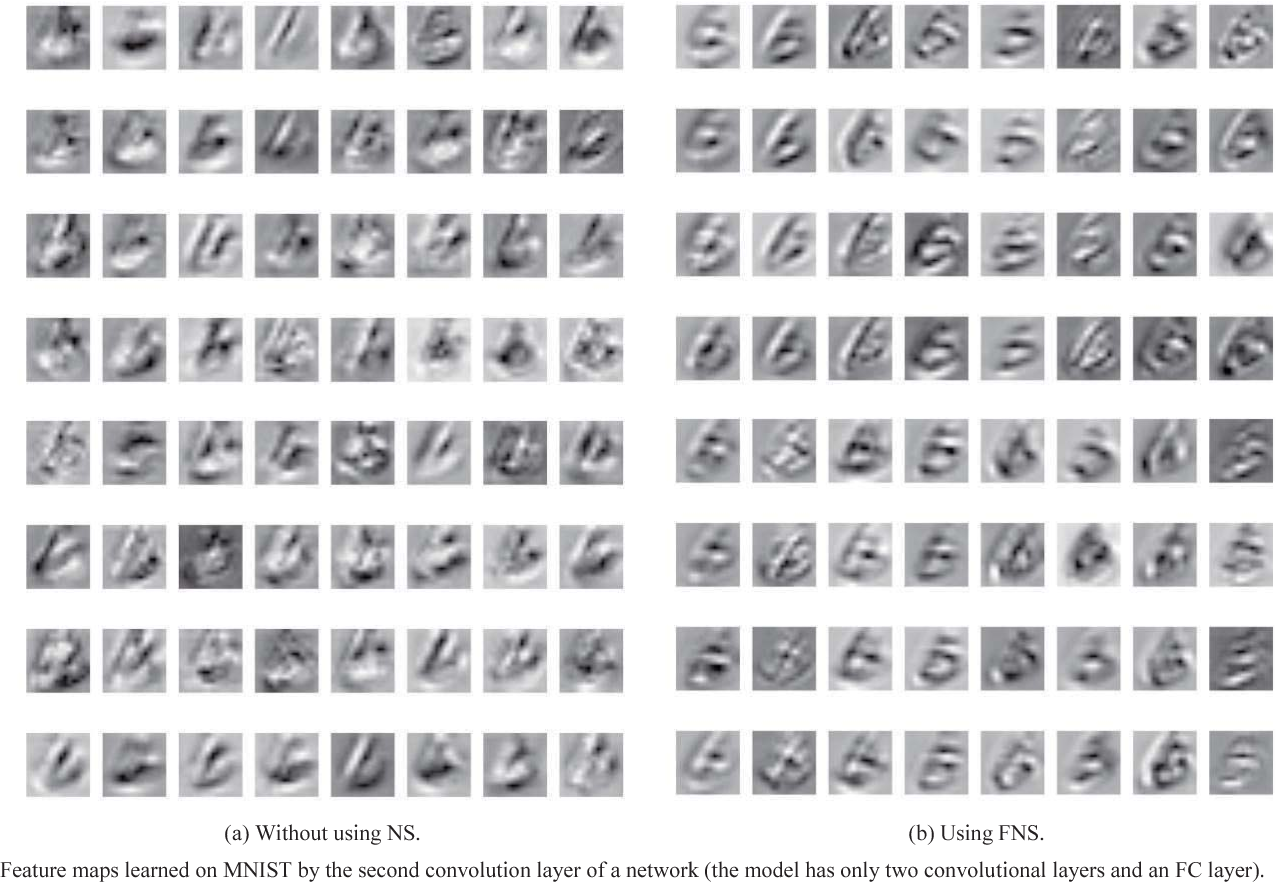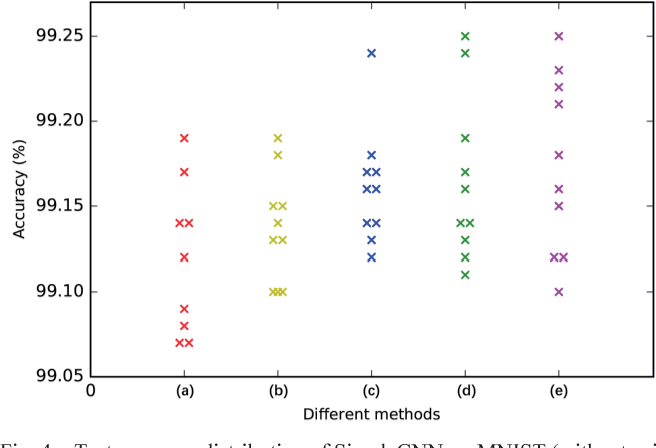Considering the use of Fully Connected (FC) layer limits the performance of Convolutional Neural Networks (CNNs), this paper develops a method to improve the coupling between the convolution layer and the FC layer by reducing the noise in Feature Maps (FMs). Our approach is divided into three steps. Firstly, we separate all the FMs into n blocks equally. Then, the weighted summation of FMs at the same position in all blocks constitutes a new block of FMs. Finally, we replicate this new block into n copies and concatenate them as the input to the FC layer. This sharing of FMs could reduce the noise in them apparently and avert the impact by a particular FM on the specific part weight of hidden layers, hence preventing the network from overfitting to some extent. Using the Fermat Lemma, we prove that this method could make the global minima value range of the loss function wider, by which makes it easier for neural networks to converge and accelerates the convergence process. This method does not significantly increase the amounts of network parameters (only a few more coefficients added), and the experiments demonstrate that this method could increase the convergence speed and improve the classification performance of neural networks.

* 7 pages, 5 figures, submitted to ICALIP 2018
Click to Read Paper and Get Code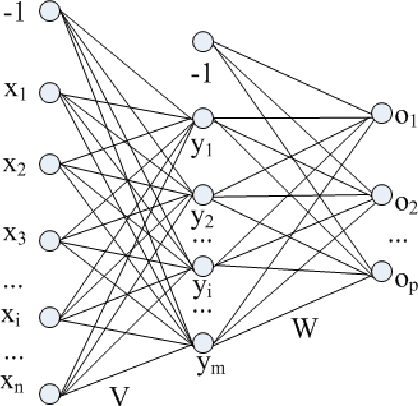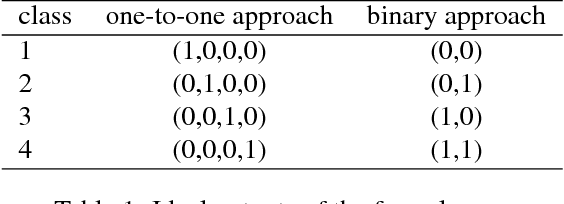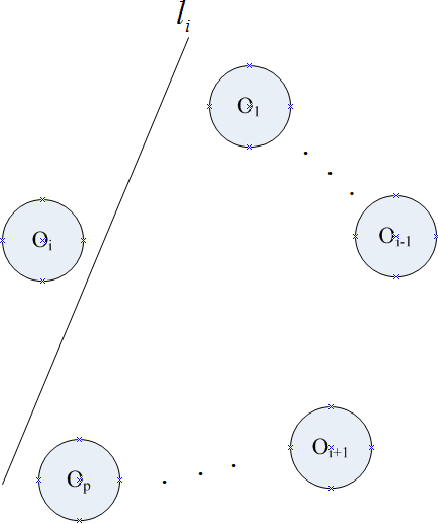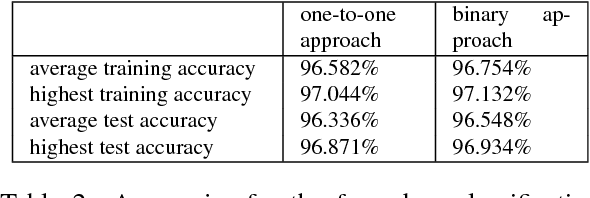Considered in this short note is the design of output layer nodes of feedforward neural networks for solving multi-class classification problems with r (bigger than or equal to 3) classes of samples. The common and conventional setting of output layer, called "one-to-one approach" in this paper, is as follows: The output layer contains r output nodes corresponding to the r classes. And for an input sample of the i-th class, the ideal output is 1 for the i-th output node, and 0 for all the other output nodes. We propose in this paper a new "binary approach": Suppose r is (2^(q minus 1), 2^q] with q bigger than or equal to 2, then we let the output layer contain q output nodes, and let the ideal outputs for the r classes be designed in a binary manner. Numerical experiments carried out in this paper show that our binary approach does equally good job as, but uses less output nodes than, the traditional one-to-one approach.

Click to Read Paper and Get Code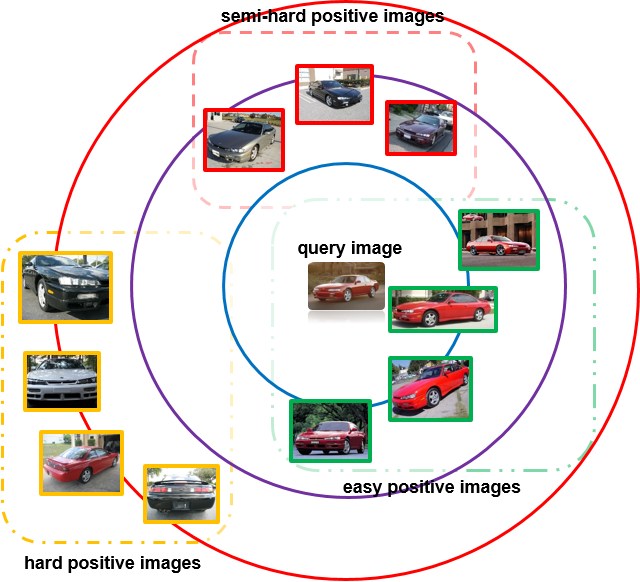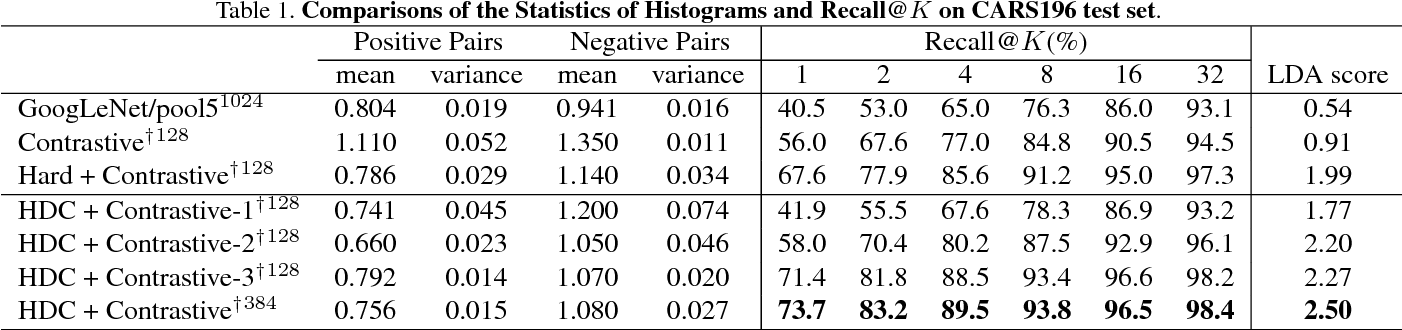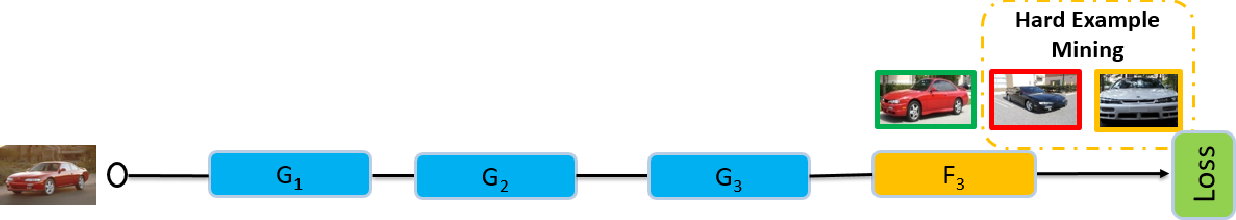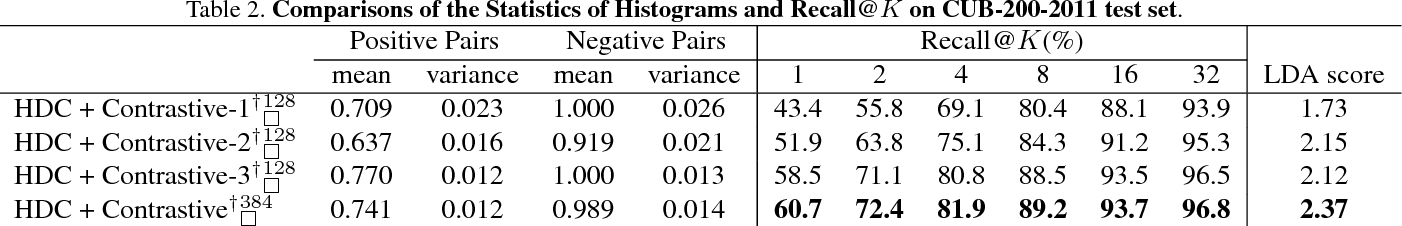Riding on the waves of deep neural networks, deep metric learning has also achieved promising results in various tasks using triplet network or Siamese network. Though the basic goal of making images from the same category closer than the ones from different categories is intuitive, it is hard to directly optimize due to the quadratic or cubic sample size. To solve the problem, hard example mining which only focuses on a subset of samples that are considered hard is widely used. However, hard is defined relative to a model, where complex models treat most samples as easy ones and vice versa for simple models, and both are not good for training. Samples are also with different hard levels, it is hard to define a model with the just right complexity and choose hard examples adequately. This motivates us to ensemble a set of models with different complexities in cascaded manner and mine hard examples adaptively, a sample is judged by a series of models with increasing complexities and only updates models that consider the sample as a hard case. We evaluate our method on CARS196, CUB-200-2011, Stanford Online Products, VehicleID and DeepFashion datasets. Our method outperforms state-of-the-art methods by a large margin.

* accepted by ICCV 2017
Click to Read Paper and Get Code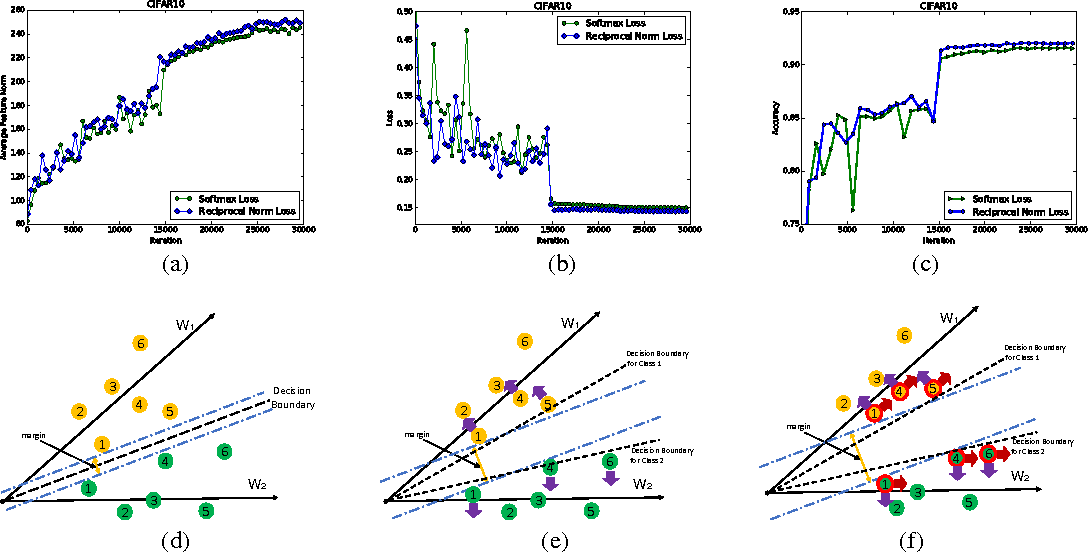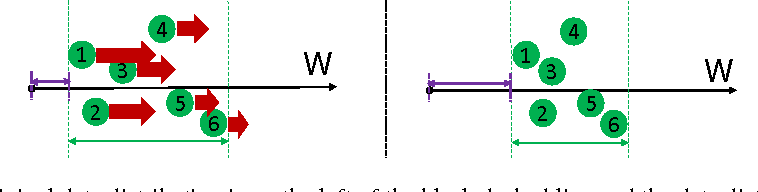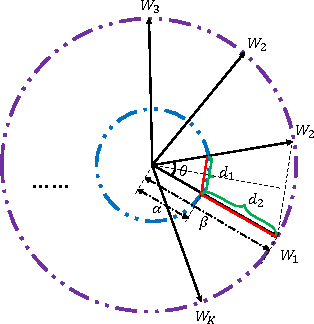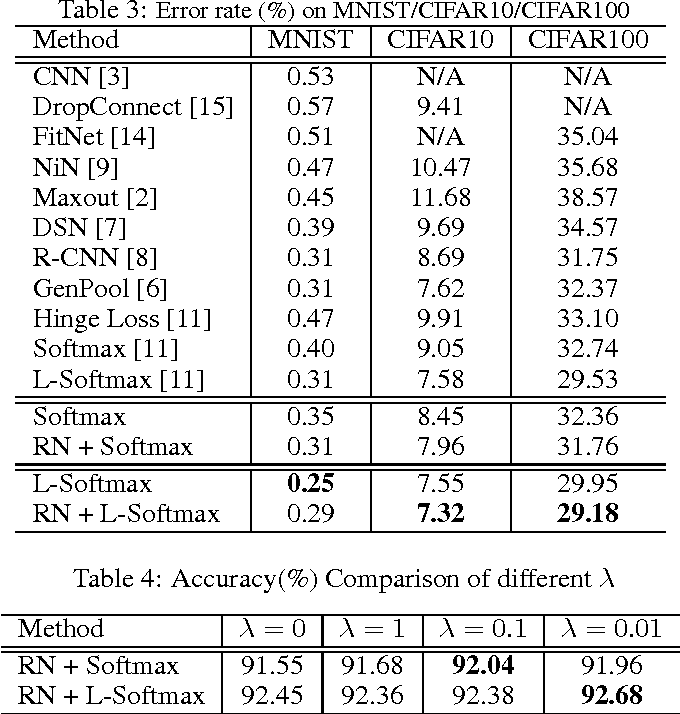Softmax loss is widely used in deep neural networks for multi-class classification, where each class is represented by a weight vector, a sample is represented as a feature vector, and the feature vector has the largest projection on the weight vector of the correct category when the model correctly classifies a sample. To ensure generalization, weight decay that shrinks the weight norm is often used as regularizer. Different from traditional learning algorithms where features are fixed and only weights are tunable, features are also tunable as representation learning in deep learning. Thus, we propose feature incay to also regularize representation learning, which favors feature vectors with large norm when the samples can be correctly classified. With the feature incay, feature vectors are further pushed away from the origin along the direction of their corresponding weight vectors, which achieves better inter-class separability. In addition, the proposed feature incay encourages intra-class compactness along the directions of weight vectors by increasing the small feature norm faster than the large ones. Empirical results on MNIST, CIFAR10 and CIFAR100 demonstrate feature incay can improve the generalization ability.

Click to Read Paper and Get Code
Evolutionary algorithms (EAs) are population-based general-purpose optimization algorithms, and have been successfully applied in various real-world optimization tasks. However, previous theoretical studies often employ EAs with only a parent or offspring population and focus on specific problems. Furthermore, they often only show upper bounds on the running time, while lower bounds are also necessary to get a complete understanding of an algorithm. In this paper, we analyze the running time of the ($\mu$+$\lambda$)-EA (a general population-based EA with mutation only) on the class of pseudo-Boolean functions with a unique global optimum. By applying the recently proposed switch analysis approach, we prove the lower bound $\Omega(n \ln n+ \mu + \lambda n\ln\ln n/ \ln n)$ for the first time. Particularly on the two widely-studied problems, OneMax and LeadingOnes, the derived lower bound discloses that the ($\mu$+$\lambda$)-EA will be strictly slower than the (1+1)-EA when the population size $\mu$ or $\lambda$ is above a moderate order. Our results imply that the increase of population size, while usually desired in practice, bears the risk of increasing the lower bound of the running time and thus should be carefully considered.

Click to Read Paper and Get Code
A large number of experimental data shows that Support Vector Machine (SVM) algorithm has obvious advantages in text classification, handwriting recognition, image classification, bioinformatics, and some other fields. To some degree, the optimization of SVM depends on its kernel function and Slack variable, the determinant of which is its parameters $\delta$ and c in the classification function. That is to say,to optimize the SVM algorithm, the optimization of the two parameters play a huge role. Ant Colony Optimization (ACO) is optimization algorithm which simulate ants to find the optimal path.In the available literature, we mix the ACO algorithm and Parallel algorithm together to find a well parameters.

* 3 pages, 2 figures, 2 tables
Click to Read Paper and Get Code
Many optimization tasks have to be handled in noisy environments, where we cannot obtain the exact evaluation of a solution but only a noisy one. For noisy optimization tasks, evolutionary algorithms (EAs), a kind of stochastic metaheuristic search algorithm, have been widely and successfully applied. Previous work mainly focuses on empirical studying and designing EAs for noisy optimization, while, the theoretical counterpart has been little investigated. In this paper, we investigate a largely ignored question, i.e., whether an optimization problem will always become harder for EAs in a noisy environment. We prove that the answer is negative, with respect to the measurement of the expected running time. The result implies that, for optimization tasks that have already been quite hard to solve, the noise may not have a negative effect, and the easier a task the more negatively affected by the noise. On a representative problem where the noise has a strong negative effect, we examine two commonly employed mechanisms in EAs dealing with noise, the re-evaluation and the threshold selection strategies. The analysis discloses that the two strategies, however, both are not effective, i.e., they do not make the EA more noise tolerant. We then find that a small modification of the threshold selection allows it to be proven as an effective strategy for dealing with the noise in the problem.

Click to Read Paper and Get Code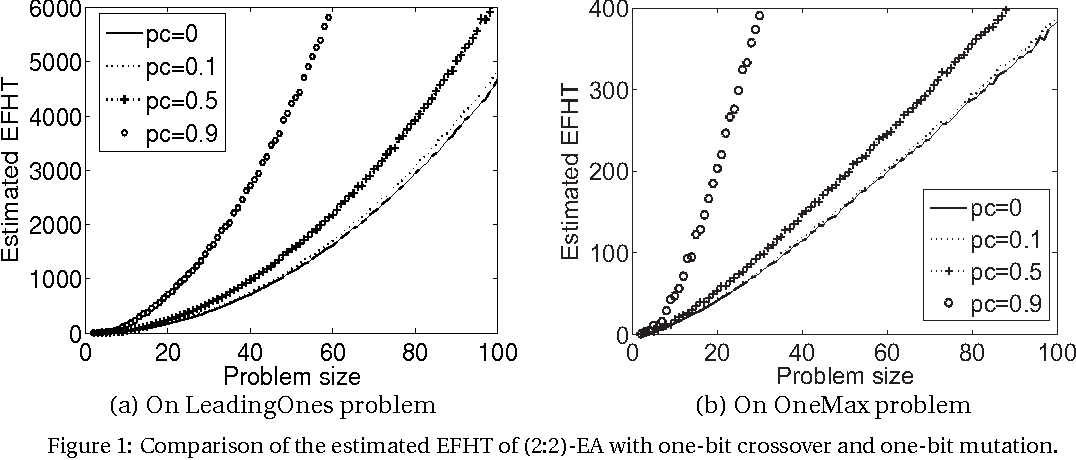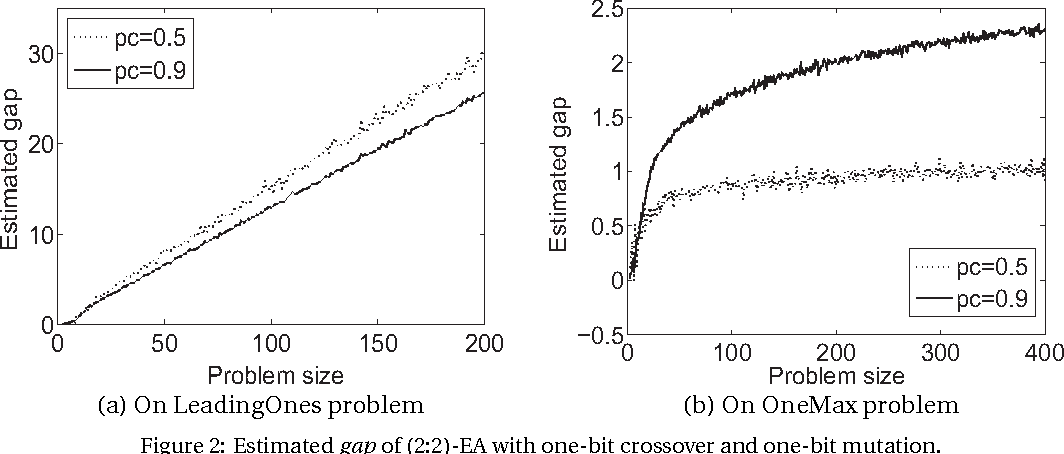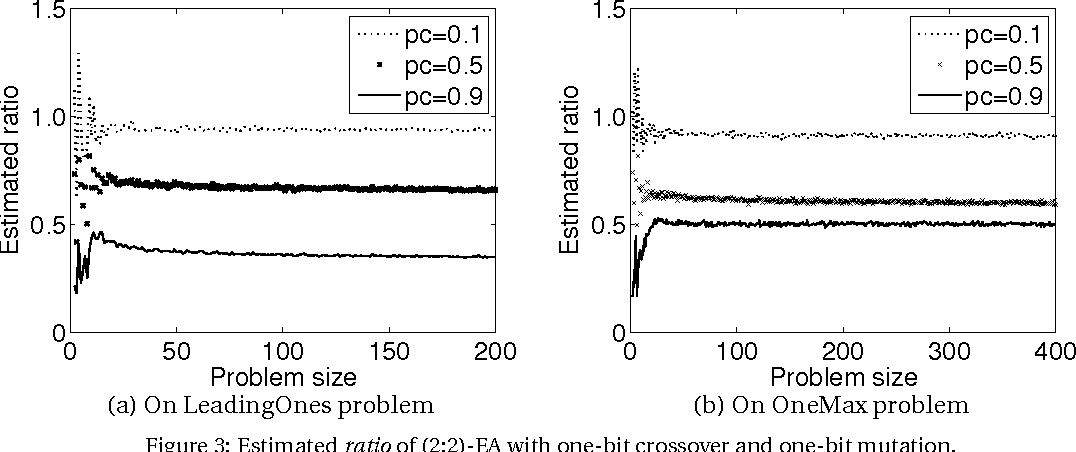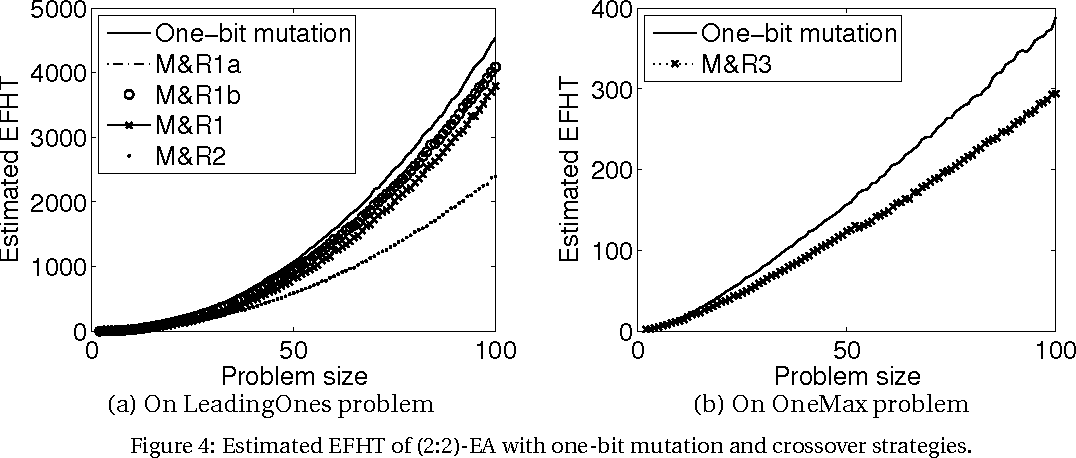Evolutionary algorithms (EAs), simulating the evolution process of natural species, are used to solve optimization problems. Crossover (also called recombination), originated from simulating the chromosome exchange phenomena in zoogamy reproduction, is widely employed in EAs to generate offspring solutions, of which the effectiveness has been examined empirically in applications. However, due to the irregularity of crossover operators and the complicated interactions to mutation, crossover operators are hard to analyze and thus have few theoretical results. Therefore, analyzing crossover not only helps in understanding EAs, but also helps in developing novel techniques for analyzing sophisticated metaheuristic algorithms. In this paper, we derive the General Markov Chain Switching Theorem (GMCST) to facilitate theoretical studies of crossover-enabled EAs. The theorem allows us to analyze the running time of a sophisticated EA from an easy-to-analyze EA. Using this tool, we analyze EAs with several crossover operators on the LeadingOnes and OneMax problems, which are noticeably two well studied problems for mutation-only EAs but with few results for crossover-enabled EAs. We first derive the bounds of running time of the (2+2)-EA with crossover operators; then we study the running time gap between the mutation-only (2:2)-EA and the (2:2)-EA with crossover operators; finally, we develop strategies that apply crossover operators only when necessary, which improve from the mutation-only as well as the crossover-all-the-time (2:2)-EA. The theoretical results are verified by experiments.

Click to Read Paper and Get Code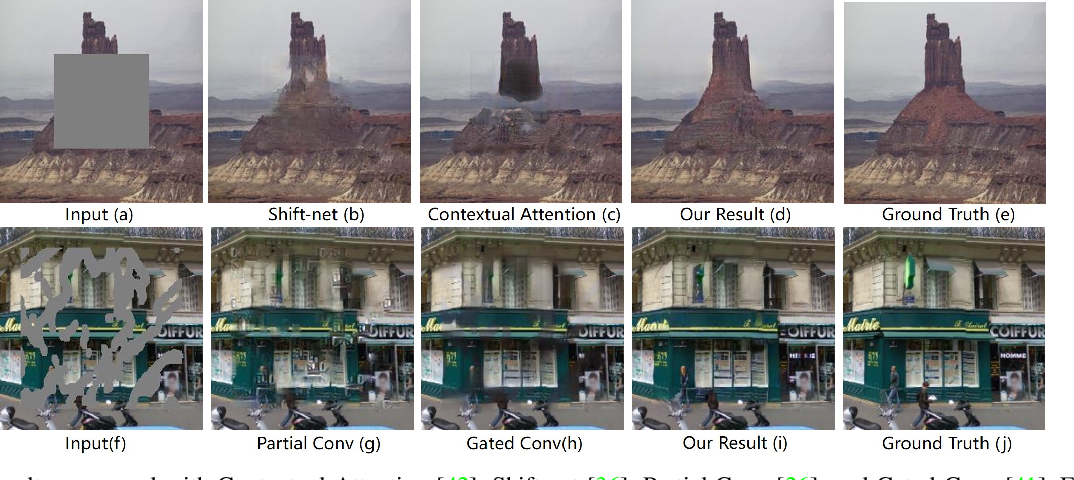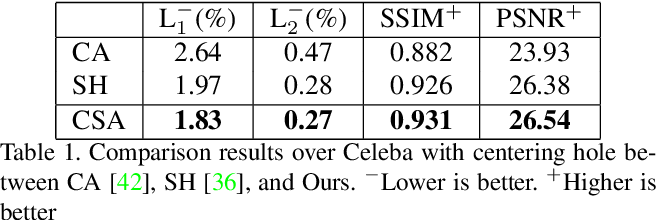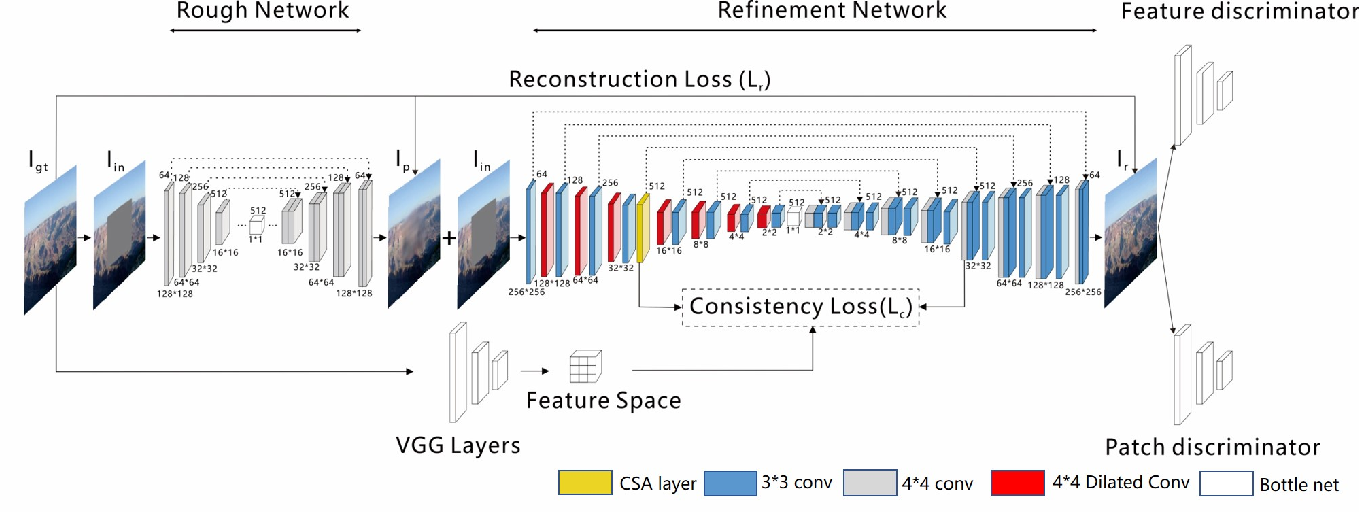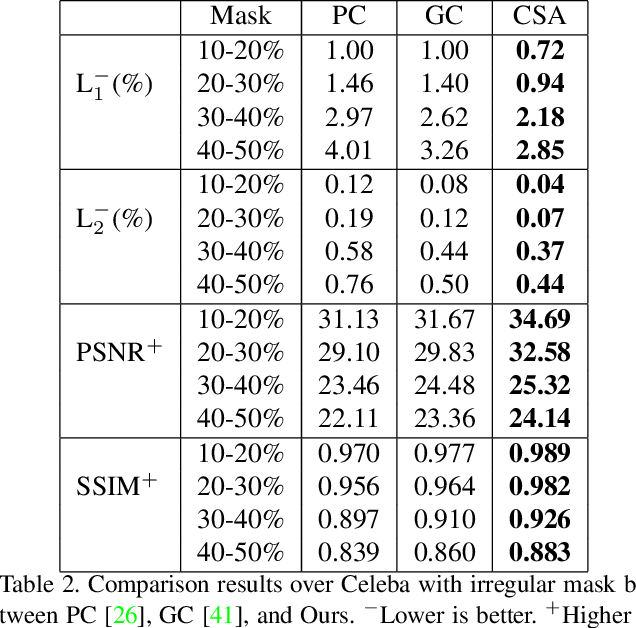The latest deep learning-based approaches have shown promising results for the challenging task of inpainting missing regions of an image. However, the existing methods often generate contents with blurry textures and distorted structures due to the discontinuity of the local pixels. From a semantic-level perspective, the local pixel discontinuity is mainly because these methods ignore the semantic relevance and feature continuity of hole regions. To handle this problem, we investigate the human behavior in repairing pictures and propose a fined deep generative model-based approach with a novel coherent semantic attention (CSA) layer, which can not only preserve contextual structure but also make more effective predictions of missing parts by modeling the semantic relevance between the holes features. The task is divided into rough, refinement as two steps and model each step with a neural network under the U-Net architecture, where the CSA layer is embedded into the encoder of refinement step. To stabilize the network training process and promote the CSA layer to learn more effective parameters, we propose a consistency loss to enforce the both the CSA layer and the corresponding layer of the CSA in decoder to be close to the VGG feature layer of a ground truth image simultaneously. The experiments on CelebA, Places2, and Paris StreetView datasets have validated the effectiveness of our proposed methods in image inpainting tasks and can obtain images with a higher quality as compared with the existing state-of-the-art approaches.

Click to Read Paper and Get Code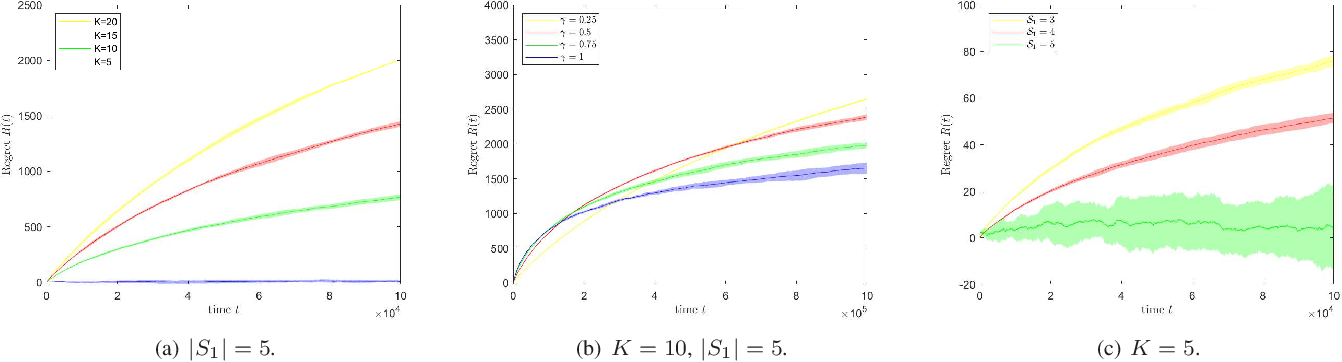In this paper, we investigate the impact of diverse user preference on learning under the stochastic multi-armed bandit (MAB) framework. We aim to show that when the user preferences are sufficiently diverse and each arm can be optimal for certain users, the O(log T) regret incurred by exploring the sub-optimal arms under the standard stochastic MAB setting can be reduced to a constant. Our intuition is that to achieve sub-linear regret, the number of times an optimal arm being pulled should scale linearly in time; when all arms are optimal for certain users and pulled frequently, the estimated arm statistics can quickly converge to their true values, thus reducing the need of exploration dramatically. We cast the problem into a stochastic linear bandits model, where both the users preferences and the state of arms are modeled as {independent and identical distributed (i.i.d)} d-dimensional random vectors. After receiving the user preference vector at the beginning of each time slot, the learner pulls an arm and receives a reward as the linear product of the preference vector and the arm state vector. We also assume that the state of the pulled arm is revealed to the learner once its pulled. We propose a Weighted Upper Confidence Bound (W-UCB) algorithm and show that it can achieve a constant regret when the user preferences are sufficiently diverse. The performance of W-UCB under general setups is also completely characterized and validated with synthetic data.

* 10 pages, 3 figures
Click to Read Paper and Get Code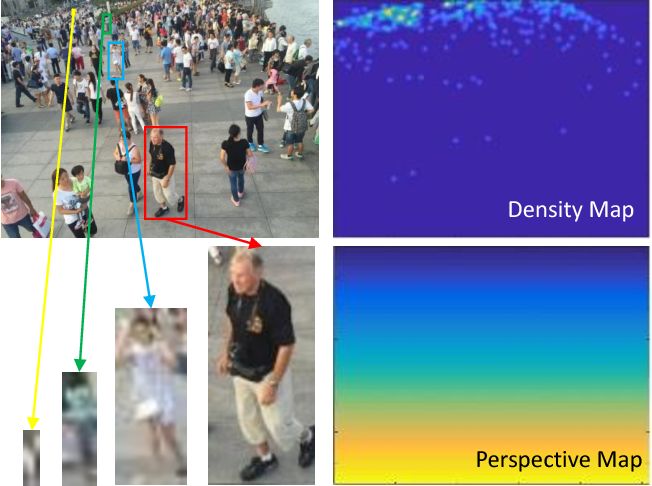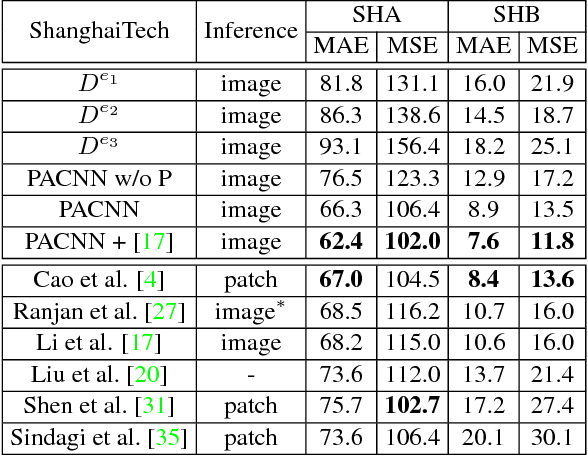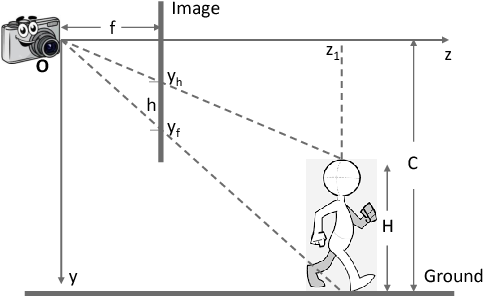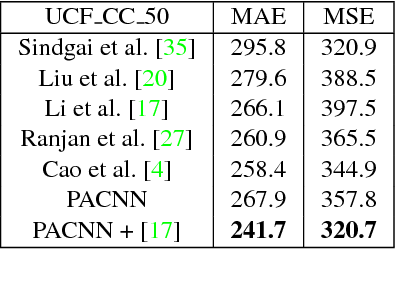Crowd counting is the task of estimating pedestrian numbers in crowd images. Modern crowd counting methods employ deep neural networks to estimate crowd counts via crowd density regressions. A major challenge of this task lies in the drastic changes of scales and perspectives in images. Representative approaches usually utilize different (large) sized filters and conduct patch-based estimations to tackle it, which is however computationally expensive. In this paper, we propose a perspective-aware convolutional neural network (PACNN) with a single backbone of small filters (e.g. 3x3). It directly predicts a perspective map in the network and encodes it as a perspective-aware weighting layer to adaptively combine the density outputs from multi-scale feature maps. The weights are learned at every pixel of the map such that the final combination is robust to perspective changes and pedestrian size variations. We conduct extensive experiments on the ShanghaiTech, WorldExpo'10 and UCF_CC_50 datasets, and demonstrate that PACNN achieves state-of-the-art results and runs as fast as the fastest.

Click to Read Paper and Get Code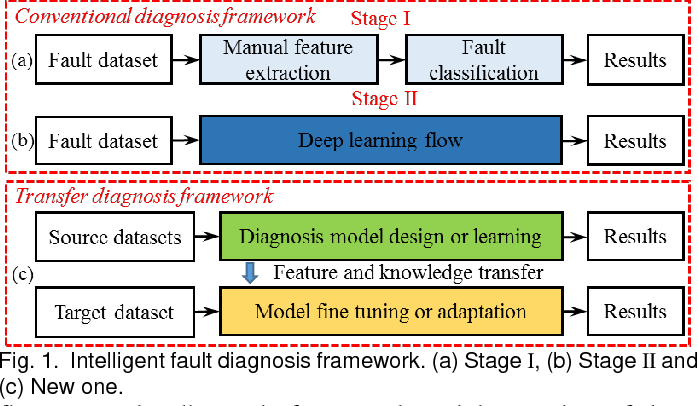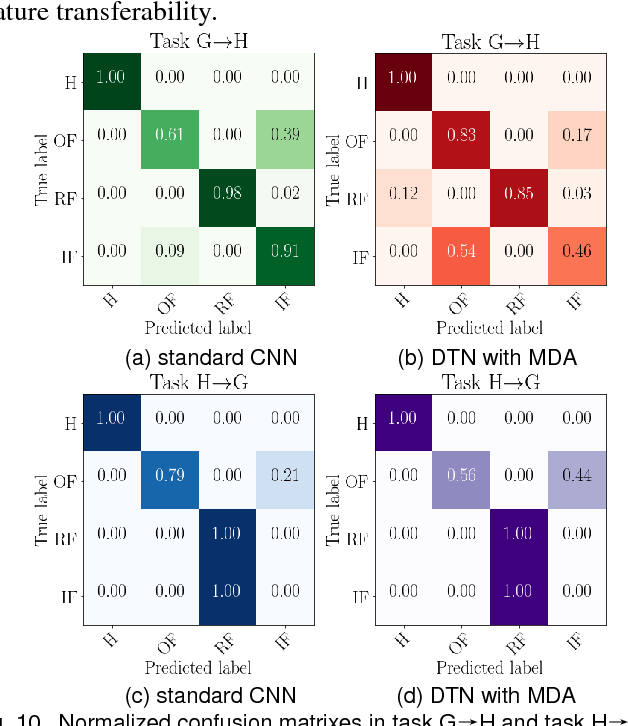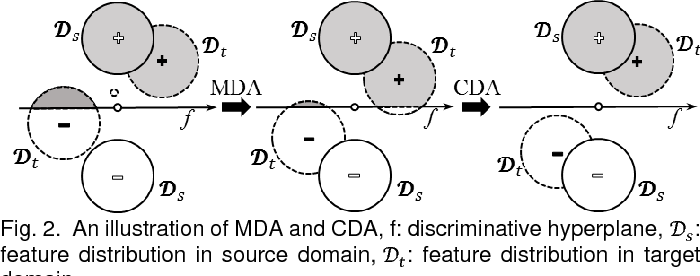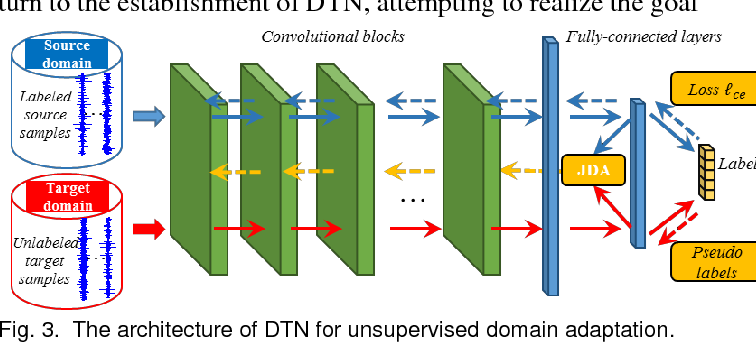In recent years, an increasing popularity of deep learning model for intelligent condition monitoring and diagnosis as well as prognostics used for mechanical systems and structures has been observed. In the previous studies, however, a major assumption accepted by default, is that the training and testing data are taking from same feature distribution. Unfortunately, this assumption is mostly invalid in real application, resulting in a certain lack of applicability for the traditional diagnosis approaches. Inspired by the idea of transfer learning that leverages the knowledge learnt from rich labeled data in source domain to facilitate diagnosing a new but similar target task, a new intelligent fault diagnosis framework, i.e., deep transfer network (DTN), which generalizes deep learning model to domain adaptation scenario, is proposed in this paper. By extending the marginal distribution adaptation (MDA) to joint distribution adaptation (JDA), the proposed framework can exploit the discrimination structures associated with the labeled data in source domain to adapt the conditional distribution of unlabeled target data, and thus guarantee a more accurate distribution matching. Extensive empirical evaluations on three fault datasets validate the applicability and practicability of DTN, while achieving many state-of-the-art transfer results in terms of diverse operating conditions, fault severities and fault types.

* 10 pages, 10 figures
Click to Read Paper and Get Code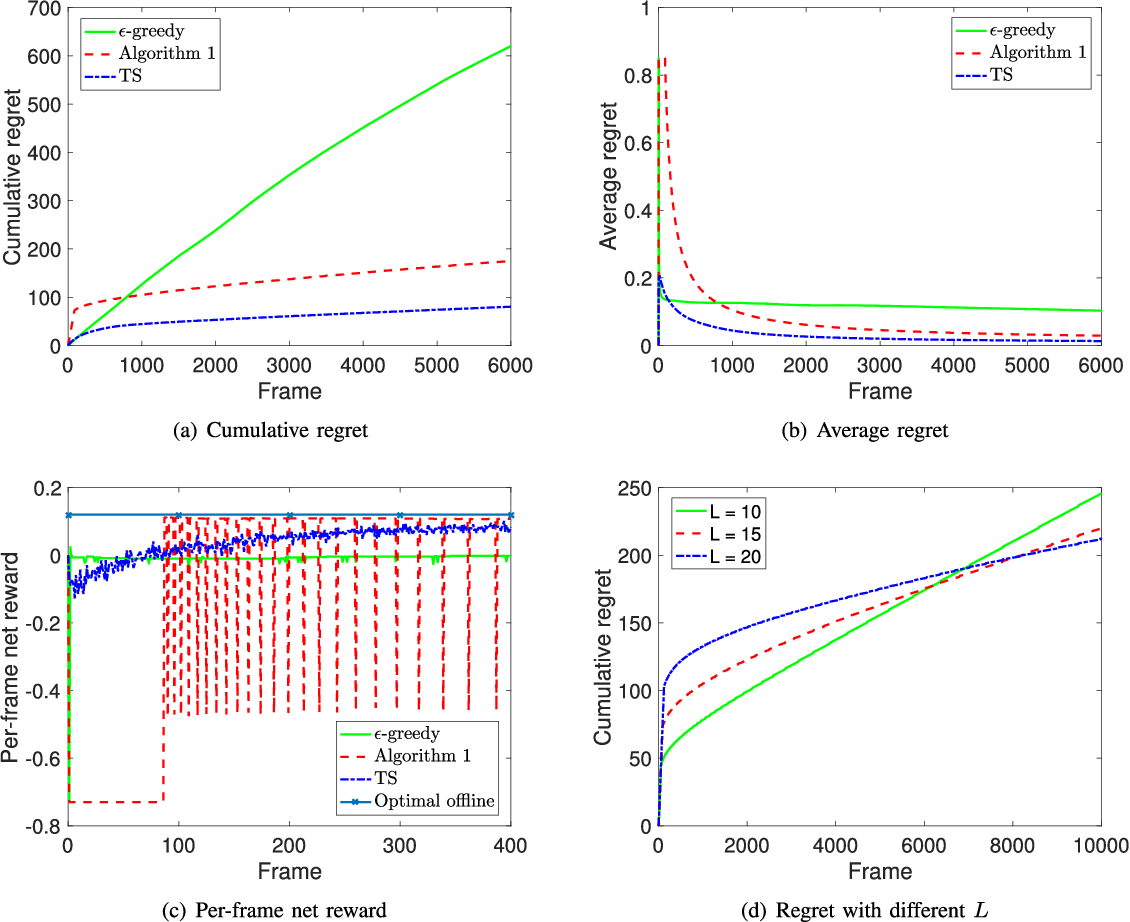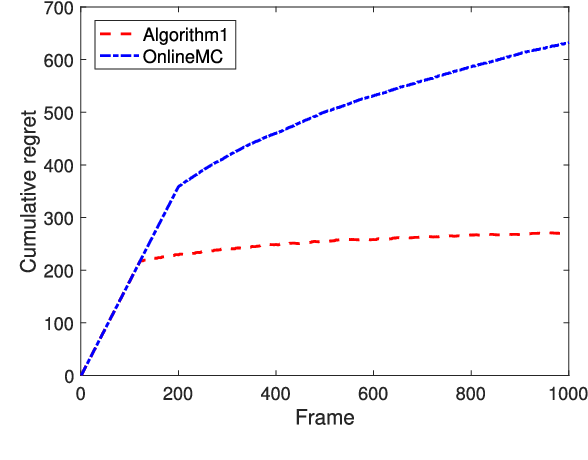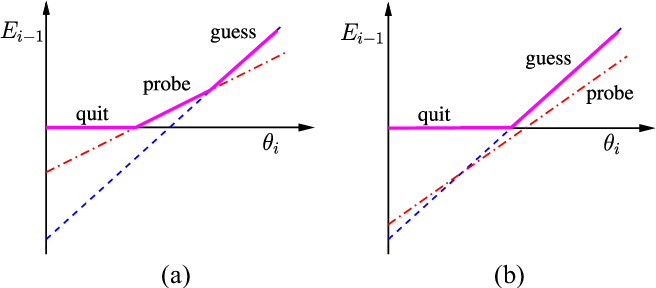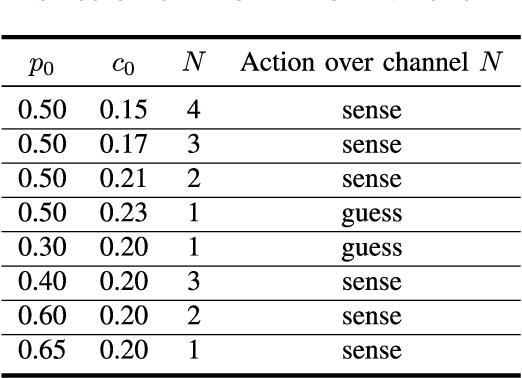In this paper, we investigate cost-aware joint learning and optimization for multi-channel opportunistic spectrum access in a cognitive radio system. We investigate a discrete time model where the time axis is partitioned into frames. Each frame consists of a sensing phase, followed by a transmission phase. During the sensing phase, the user is able to sense a subset of channels sequentially before it decides to use one of them in the following transmission phase. We assume the channel states alternate between busy and idle according to independent Bernoulli random processes from frame to frame. To capture the inherent uncertainty in channel sensing, we assume the reward of each transmission when the channel is idle is a random variable. We also associate random costs with sensing and transmission actions. Our objective is to understand how the costs and reward of the actions would affect the optimal behavior of the user in both offline and online settings, and design the corresponding opportunistic spectrum access strategies to maximize the expected cumulative net reward (i.e., reward-minus-cost). We start with an offline setting where the statistics of the channel status, costs and reward are known beforehand. We show that the the optimal policy exhibits a recursive double threshold structure, and the user needs to compare the channel statistics with those thresholds sequentially in order to decide its actions. With such insights, we then study the online setting, where the statistical information of the channels, costs and reward are unknown a priori. We judiciously balance exploration and exploitation, and show that the cumulative regret scales in O(log T). We also establish a matched lower bound, which implies that our online algorithm is order-optimal. Simulation results corroborate our theoretical analysis.

* 12 pages, 6 figures
Click to Read Paper and Get Code
Numerous single-image super-resolution algorithms have been proposed in the literature, but few studies address the problem of performance evaluation based on visual perception. While most super-resolution images are evaluated by fullreference metrics, the effectiveness is not clear and the required ground-truth images are not always available in practice. To address these problems, we conduct human subject studies using a large set of super-resolution images and propose a no-reference metric learned from visual perceptual scores. Specifically, we design three types of low-level statistical features in both spatial and frequency domains to quantify super-resolved artifacts, and learn a two-stage regression model to predict the quality scores of super-resolution images without referring to ground-truth images. Extensive experimental results show that the proposed metric is effective and efficient to assess the quality of super-resolution images based on human perception.

* Accepted by Computer Vision and Image Understanding
Click to Read Paper and Get Code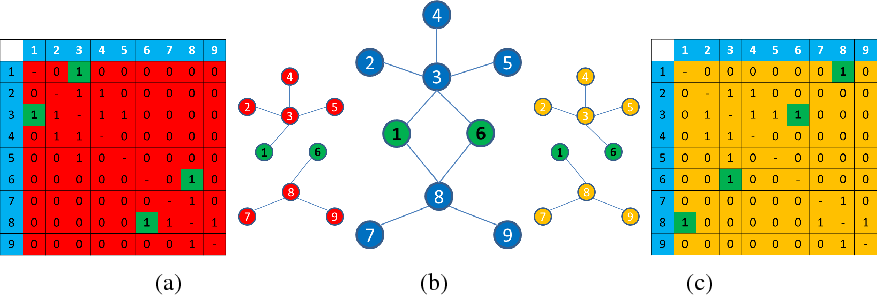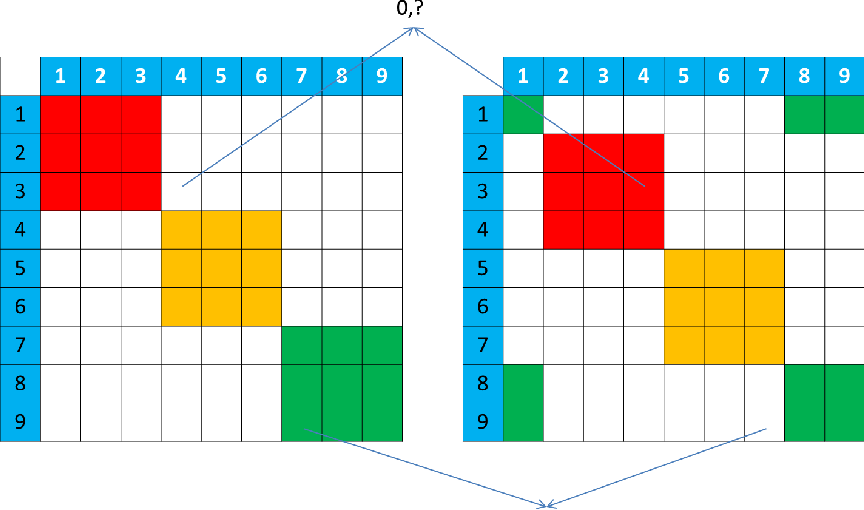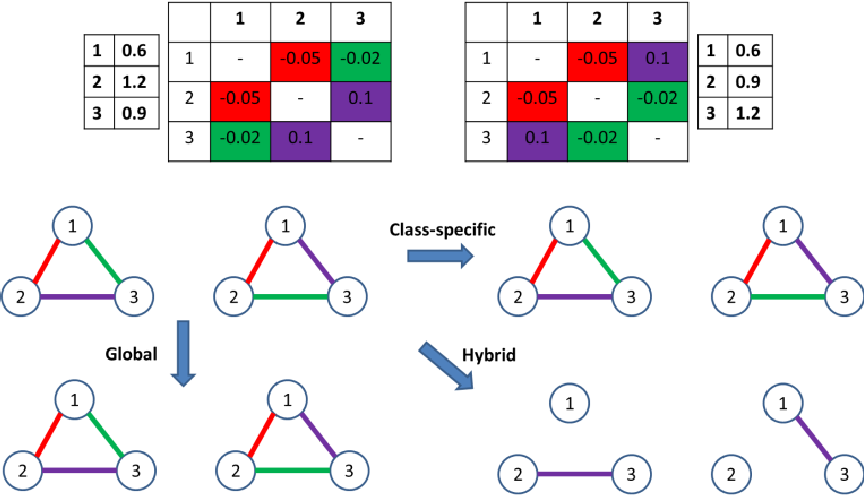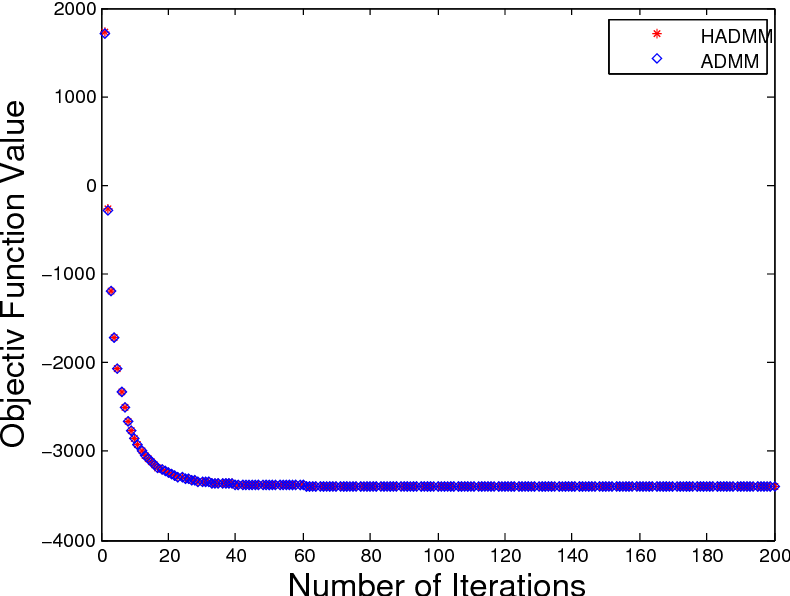This paper considers the problem of estimating multiple related Gaussian graphical models from a $p$-dimensional dataset consisting of different classes. Our work is based upon the formulation of this problem as group graphical lasso. This paper proposes a novel hybrid covariance thresholding algorithm that can effectively identify zero entries in the precision matrices and split a large joint graphical lasso problem into small subproblems. Our hybrid covariance thresholding method is superior to existing uniform thresholding methods in that our method can split the precision matrix of each individual class using different partition schemes and thus split group graphical lasso into much smaller subproblems, each of which can be solved very fast. In addition, this paper establishes necessary and sufficient conditions for our hybrid covariance thresholding algorithm. The superior performance of our thresholding method is thoroughly analyzed and illustrated by a few experiments on simulated data and real gene expression data.

Click to Read Paper and Get Code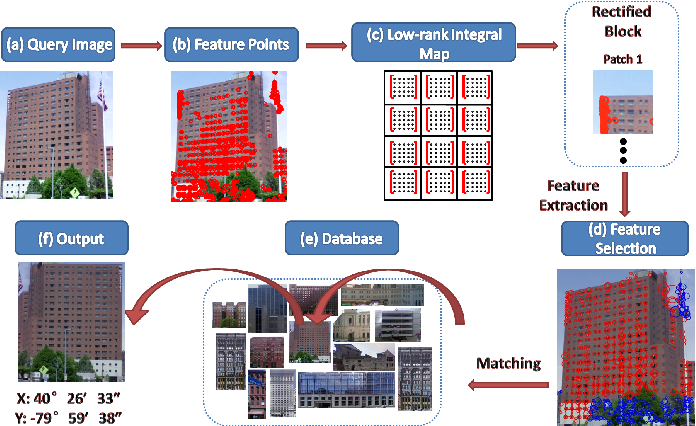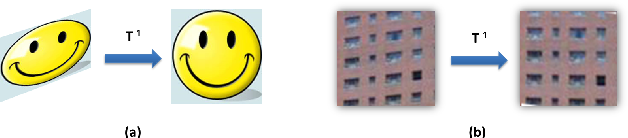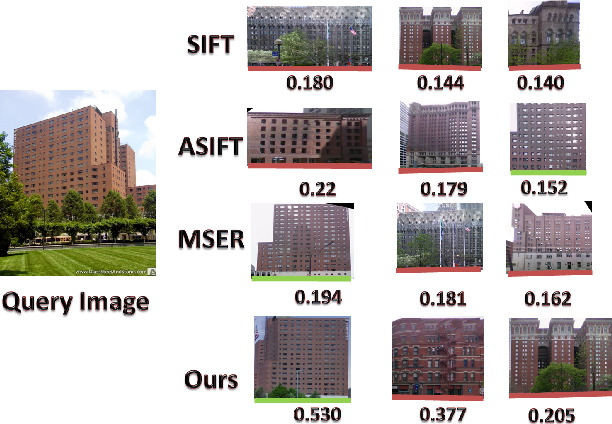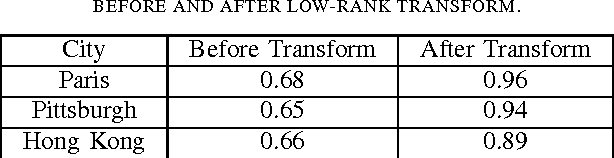In this paper, we present a novel affine-invariant feature based on SIFT, leveraging the regular appearance of man-made objects. The feature achieves full affine invariance without needing to simulate over affine parameter space. Low-rank SIFT, as we name the feature, is based on our observation that local tilt, which are caused by changes of camera axis orientation, could be normalized by converting local patches to standard low-rank forms. Rotation, translation and scaling invariance could be achieved in ways similar to SIFT. As an extension of SIFT, our method seeks to add prior to solve the ill-posed affine parameter estimation problem and normalizes them directly, and is applicable to objects with regular structures. Furthermore, owing to recent breakthrough in convex optimization, such parameter could be computed efficiently. We will demonstrate its effectiveness in place recognition as our major application. As extra contributions, we also describe our pipeline of constructing geotagged building database from the ground up, as well as an efficient scheme for automatic feature selection.

Click to Read Paper and Get Code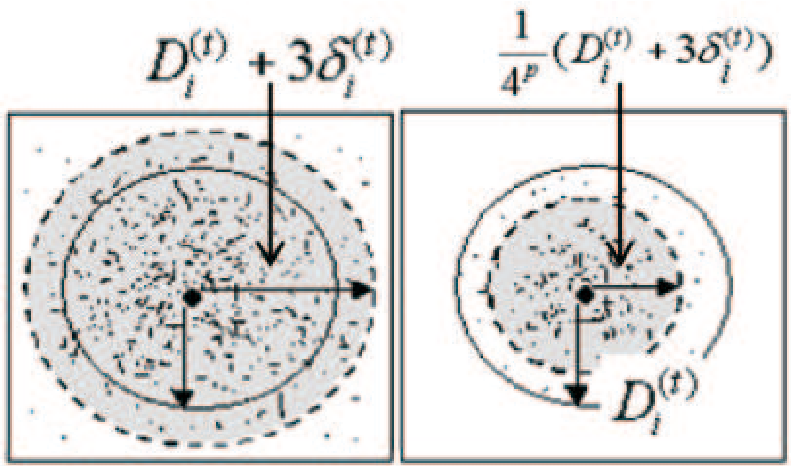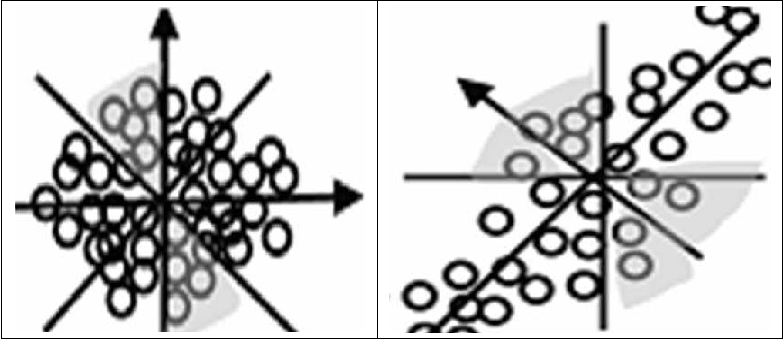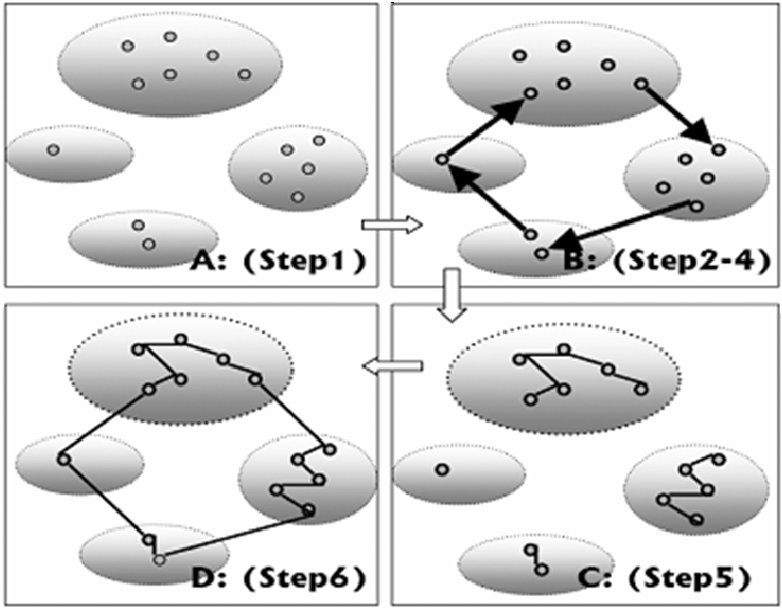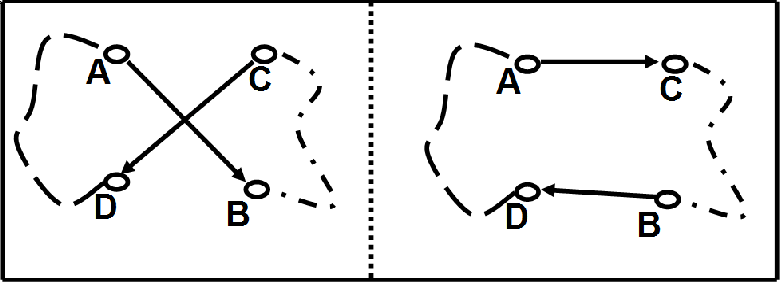Ant Colony Optimization (ACO) has time complexity O(t*m*N*N), and its typical application is to solve Traveling Salesman Problem (TSP), where t, m, and N denotes the iteration number, number of ants, number of cities respectively. Cutting down running time is one of study focuses, and one way is to decrease parameter t and N, especially N. For this focus, the following method is presented in this paper. Firstly, design a novel clustering algorithm named Special Local Clustering algorithm (SLC), then apply it to classify all cities into compact classes, where compact class is the class that all cities in this class cluster tightly in a small region. Secondly, let ACO act on every class to get a local TSP route. Thirdly, all local TSP routes are jointed to form solution. Fourthly, the inaccuracy of solution caused by clustering is eliminated. Simulation shows that the presented method improves the running speed of ACO by 200 factors at least. And this high speed is benefit from two factors. One is that class has small size and parameter N is cut down. The route length at every iteration step is convergent when ACO acts on compact class. The other factor is that, using the convergence of route length as termination criterion of ACO and parameter t is cut down.

* 21 pages, 5figures
Click to Read Paper and Get Code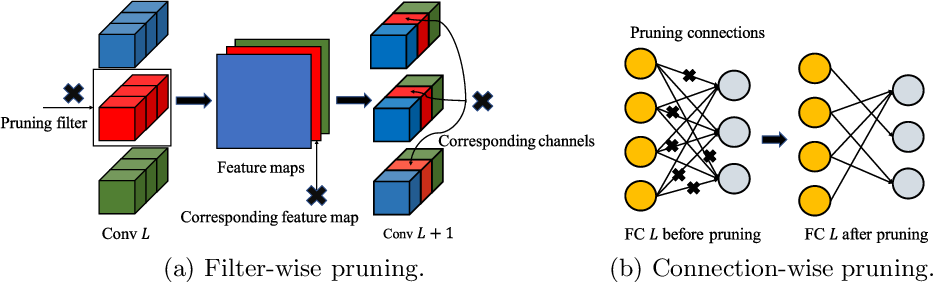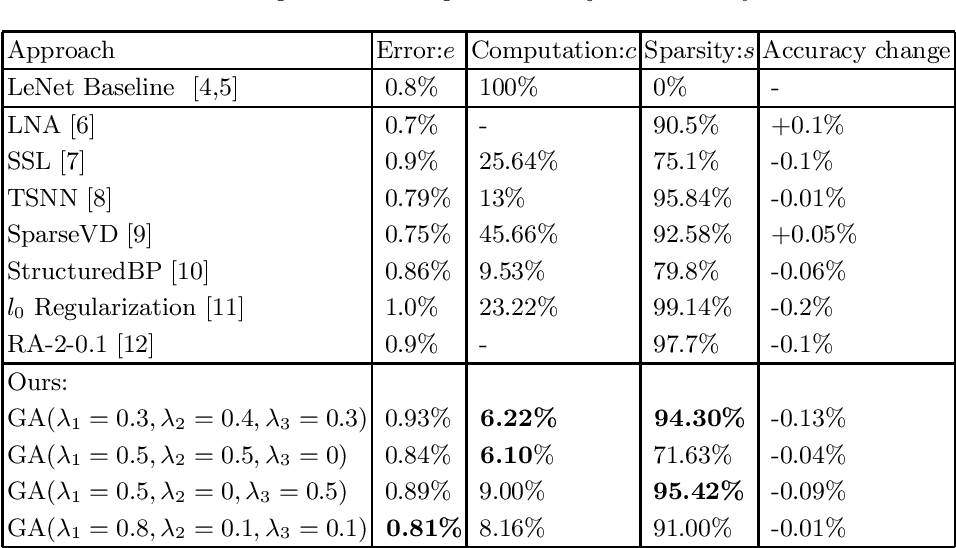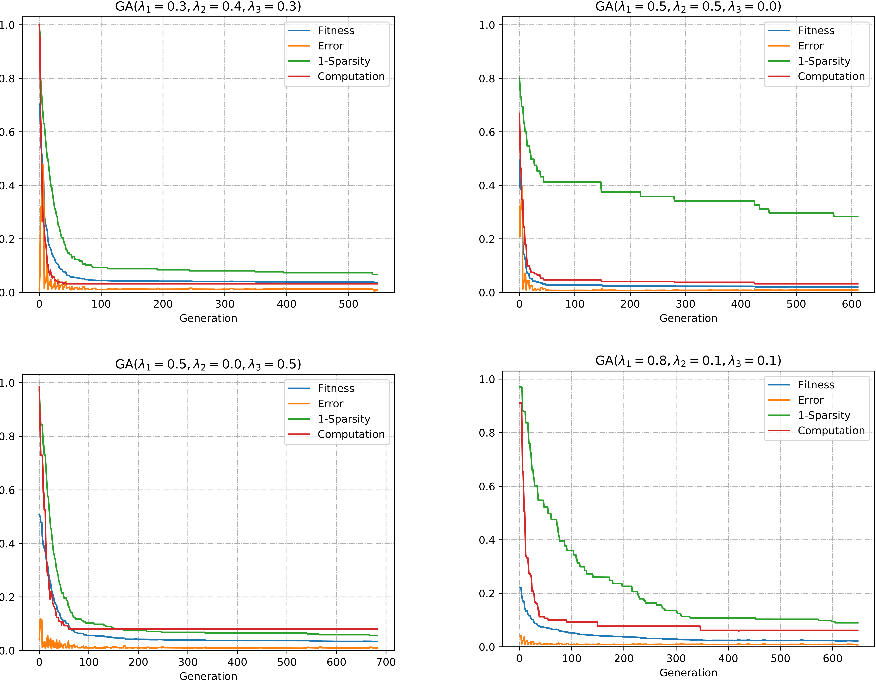In this work, we propose a heuristic genetic algorithm (GA) for pruning convolutional neural networks (CNNs) according to the multi-objective trade-off among error, computation and sparsity. In our experiments, we apply our approach to prune pre-trained LeNet across the MNIST dataset, which reduces 95.42% parameter size and achieves 16$\times$ speedups of convolutional layer computation with tiny accuracy loss by laying emphasis on sparsity and computation, respectively. Our empirical study suggests that GA is an alternative pruning approach for obtaining a competitive compression performance. Additionally, compared with state-of-the-art approaches, GA is capable of automatically pruning CNNs based on the multi-objective importance by a pre-defined fitness function.

* 6 pages,4 figures,under review as a conference paper at ICANN 2019
Click to Read Paper and Get Code